Include Top

ARIMA Forecast

1. Open Chemical Process Concentration – Series A.xlsx (Sheet 1 tab). This is the Series A data from Box and Jenkins, a set of 197 concentration values from a chemical process taken at two-hour intervals. See the Run Chart, ACF/PACF Plots, Spectral Density Plot and Seasonal Trend Decomposition Plots for this data.
2. Click SigmaXL > Time Series Forecasting > ARIMA Forecast > Forecast. Ensure that the entire data table is selected. If not, check Use Entire Data Table. Click Next.
3. Select Concentration, click Numeric Data Variable (Y) >>. Check Display ACF/PACF/LB Plots and Display Residual Plots. Leave Specify Model Periods, Seasonal Frequency and Box-Cox Transformation unchecked. We will use the default No. of Forecast Periods = 24 and Prediction Interval = 95.0 %.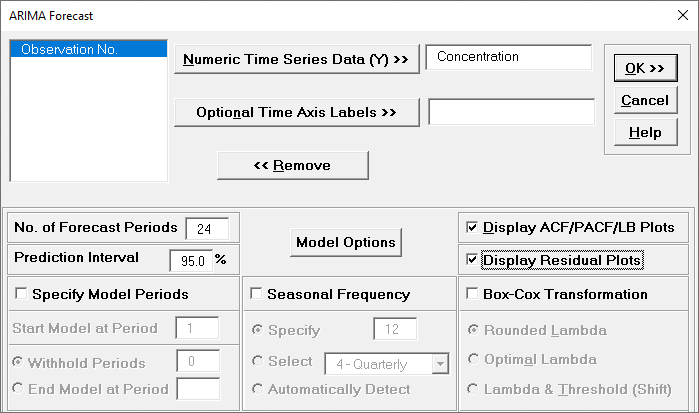Optional Time Axis Labels will be displayed on the forecast chart time axis. If used, dates for the forecast periods should also be included, otherwise the time axis will be blank for the forecast periods.

No. of Forecast Periods are the number of time series values to be predicted (forecast horizon). The most accurate forecast will be for the first predicted value (one-step-ahead).

Prediction Interval % is the confidence level for the individual predictions. For example, a 95% prediction interval contains a range of values which should include the actual future value with 95% probability. The interval will get larger the further out you predict.

Model Options opens another dialog which allows you to set automatic options or to specify a model.

Display ACF/PACF/LB option will produce ACF and PACF plots for the raw data as well as for the model residuals. The LB plot is a plot of Ljung-Box test P-Values for various lags and is used to determine if a group of autocorrelations are significant, (i.e., the autocorrelations do not come from a white noise series).

Display Residual Plots will produce a table of model residuals and the usual model residual plots: histogram, normal probability plot, residuals versus data order, and residuals vs forecast value. Note that if a Box-Cox transformation is applied, the residuals are transformed so will not be equal to forecast - actual.

Specify Model Periods are used to specify a start period, end period or withhold sample. The withheld data is not used in model estimation, so this is very useful for model validation and comparison. This will be used in a later example.

Seasonal Frequency and Box-Cox Transformation will be used in a later example.

4. Click Model Options.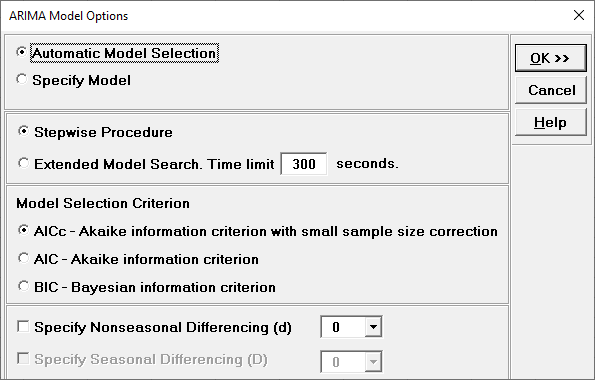Automatic Model Selection will be used later. It is the default selection.

Stepwise Procedure selects the use of the stepwise method.

Extended Model Search is described above. The Time limit may need to be increased for seasonal models with high seasonal frequency and/or large number of observations.

Model Selection Criterion is the information criterion metric to be used in automatic model selection. AICc is the default selection.

Specify Nonseasonal Differencing (d) = 0, 1, or 2, overrides the automatic nonseasonal differencing. This is useful to compare models for borderline cases that are “nearly nonstationary” (see Box and Jenkins).

Specify Seasonal Differencing (D) = 0 or 1, overrides the automatic seasonal differencing. It is greyed out here because Seasonal Frequency was unchecked in the previous dialog.

• Clicking OK accepts the settings and returns you to the previous dialog. Clicking Cancel will cancel any changes and return you to the previous dialog.

5. Select Specify Model. Specify Nonseasonal Order I – Integrated/Differencing (d) = 1 and MA – Moving Average (q) = 1. Leave Include Constant unchecked.Specify Model allows you to manually specify the Nonseasonal Order and Seasonal Order values and option for Include Constant (Mean if d + D = 0; Trend/Drift if d or D = 1). If d + D = 0, then the constant term is the mean; if d or D = 1, then the constant term is a Trend; if d + D > 1, then the constant term is quadratic or higher – this is not recommended.

Seasonal Order is greyed out because Seasonal Frequency was unchecked in the previous dialog.

The specified ARIMA (0,1,1) is equivalent to a simple exponential smoothing model (with slight differences due to estimation of the initial value).

6. Click OK to return to the ARIMA Forecast dialog. Click OK. The ARIMA forecast report is given:As expected, this is very similar to the exponential smoothing forecast chart that was produced using the Simple Exponential Smoothing with Additive Errors (A, N, N) – Exponentially Weighted Moving Average (EWMA) model. The initial in-sample predicted value for ARIMA is slightly different and starts at the second time period due to differencing.

7. Scroll down to view the ARIMA Model header: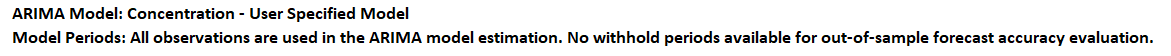If we had checked Specify Model Periods in the main dialog, the start, end or withhold selection would be summarized here as well.

8. The ARIMA Model Summary is given as:This is a summary of model information: ARIMA (0,1,1) with no constant and no predictors. Seasonal Frequency = 1 (nonseasonal); Model Selection Criterion = “Specified” because the model was user specified; and Box-Cox Transformation = “N/A” because Box-Cox Transformation was unchecked.

9. The Parameter Estimates are: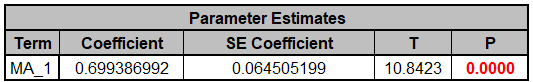The MA_1 parameter coefficient value is approximately equal to 1 – alpha = 1 - 0.2948 = 0.7052 in Exponential Smoothing Parameter Estimates. The slight difference is due to estimation of the initial value.

ARIMA Parameter Estimates include significance tests; P-Values < .05 are significant and highlighted in red. This may be useful for model refinement with multiple predictors (and will be demonstrated later). Note that for AR/MA model order selection, minimum AICc should be used, rather than significance tests (see Kostenko, A.V. and Hyndman, R.J.).

10. The ARIMA Model Statistics are:Degrees of freedom (DF) = n – 2 (1 term in the model, 1 order of differencing).

Comparing to the Exponential Smoothing Model Statistics, we see that the StDev and Variance are approximately equal, but the Log-Likelihood, AICc, AIC and BIC are very different. This is due to different formulas being used in the Likelihood function. You cannot use Information Criteria to compare ARIMA and Exponential Smooth models to determine which model has the best fit.

11. The In-Sample Forecast Accuracy metrics are:MASE is less than one, so it is a better forecast than would be obtained from a naïve forecast (set all forecasts to be the value of the last observation).

Comparing to the Exponential Smoothing Exponential Smoothing In-Sample Forecast Accuracy metrics, as expected, we see the forecast errors are approximately the same. Note that we lose one observation (N=196) since we do not have a predicted value at time period = 1 due to differencing.

12. The Forecast Table is given as:These are the same forecast and prediction interval values displayed in the Forecast Chart but provided for further analysis or charting. If Withhold Periods are specified, the Withhold Data will be displayed as well.

13. Click on the ARIMA ACF PACF LB sheet to view the ACF/PACF/LB Plots:These are approximately the same as what we obtained previously with Exponential Smoothing ACF/PACF/LB Plots. We can see that all of the autocorrelation has been removed by the exponential smoothing model (with the exception of lag 15 in the PACF), so this is a good fit to the time series data.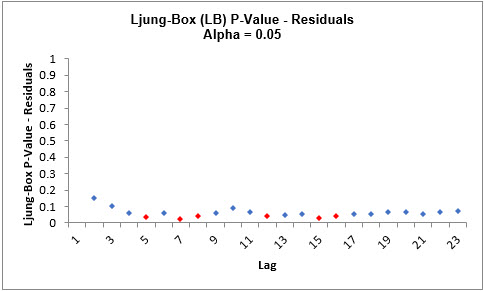The LB plot is a plot of Ljung-Box test P-Values for various lags and is used to determine if a group of autocorrelations are significant, (i.e., the autocorrelations do not come from a white noise series).

The red P-Values are significant (alpha=.05) and the blue P-Values are not significant. It is desirable that all P-Values be blue. The ACF/PACF plots indicated that almost all of the correlation has been accounted for in the model, but the Ljung-Box plot shows that some significant autocorrelation still remains - so the model can potentially be improved. This does not mean that the model is a bad model, it can still be very useful for prediction purposes, but the prediction intervals may not provide accurate coverage.

There does appear to be fewer significant P-Values than we obtained previously with the Exponential Smoothing LB plot, but this may not be a practical difference, given the similarity of all the other statistics.

14. Click on the ARIMA Residuals sheet to view the Residual Plots: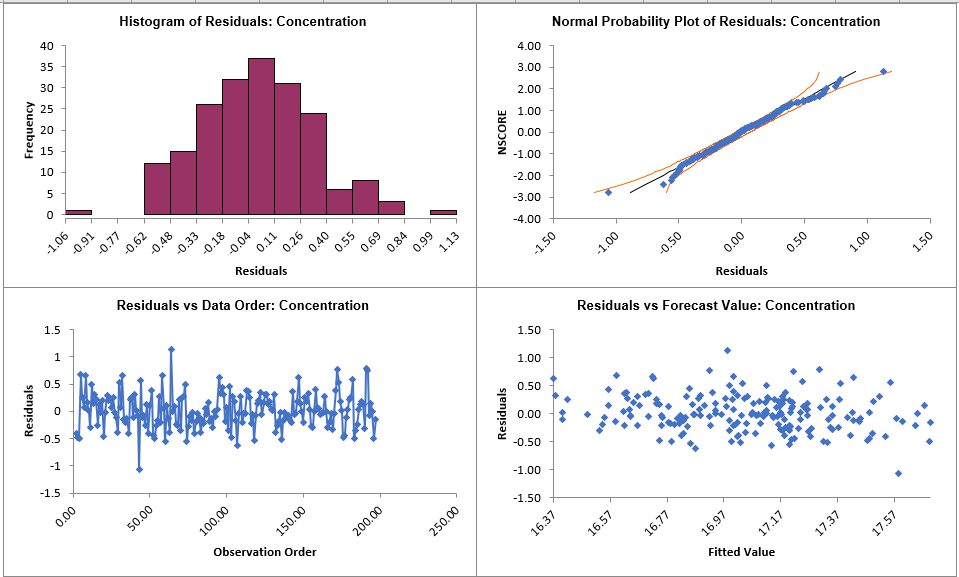The residuals are approximately normally distributed, with a roughly straight line on the normal probability plot.

There are no obvious extreme outliers or patterns in the charts. Later, we will apply a control chart to the residuals to formally test for significant outliers or assignable causes. These are approximately the same as what we obtained previously with Exponential Smoothing Residual Plots.

15. Now we will rerun ARIMA Forecast on the Concentration data with Automatic Model Selection and Specify Withhold Periods. Click Recall SigmaXL Dialog menu or press F3 to recall last dialog. Check Specify Model Periods. Set Withhold Periods = 24. We will use the default Withhold Forecast Type: One-Step-Ahead with Prediction Interval at: Start of Withhold.Specify Model Periods option allows you to specify the start and end periods used in automatic model identification and parameter estimation. Typically, Start Model at Period is kept = 1 and Withhold Periods specifies the number of periods to be withheld for out-of-sample testing. End Model at Period specifies the end period, so the withhold sample size would be: total number of observations – end period.

Withhold Forecast Type: One-Step-Ahead will exclude the withhold sample from automatic model identification and parameter estimation, but uses the withhold data to update the predicted one-step ahead forecast. This is useful to assess forecast error when you only care about the short-term one-step ahead prediction.

Withhold Forecast Type: One-Step-Ahead with Prediction Interval at: Start of Withhold will display the prediction interval for the duration of the withhold sample. Note that the length of the prediction interval is determined by the number of withhold periods, so overrides the specified No. of Forecast Periods.

Withhold Forecast Type: One-Step-Ahead with Prediction Interval at: End of Withhold will display the prediction interval at the end of the withhold sample. The length of the prediction interval is determined by the specified No. of Forecast Periods.

Include in Residuals will treat the one-step-ahead forecast errors as residuals (even though they were not part of the model estimation process) and will be included in the ACF/PACF/LB Residual Plots along with the Residuals report and graphs. Typically, this is kept unchecked.

Withhold Forecast Type: Multi-Step-Ahead with Prediction Interval at Start of Withhold will exclude the withhold sample from automatic model identification and parameter estimation and does not use the withhold data to update the predicted one-step ahead forecast. This is useful to assess forecast error when you are interested in a long-term forecast window (horizon). The prediction interval will be displayed for the duration of the withhold sample. Note that the length of the prediction interval is determined by the number of withhold periods, so overrides the specified No. of Forecast Periods. These forecast errors are not included in ACF/PACF/LB Residual Plots or the Residuals report and graphs.

16. Click Model Options. Select Automatic Model Selection. We will use the defaults: Stepwise Procedure and Model Selection Criterion: AICc – Akaike information criterion with small sample size correction, leave Specify Nonseasonal Differencing (d) unchecked.Tip: When using Recall SigmaXL Dialog and if there are no changes to the Model Option settings, the previous settings will be used. It is not necessary to repeat this step.

17. Click OK to return to the ARIMA Forecast dialog. Click OK. The ARIMA forecast report is given: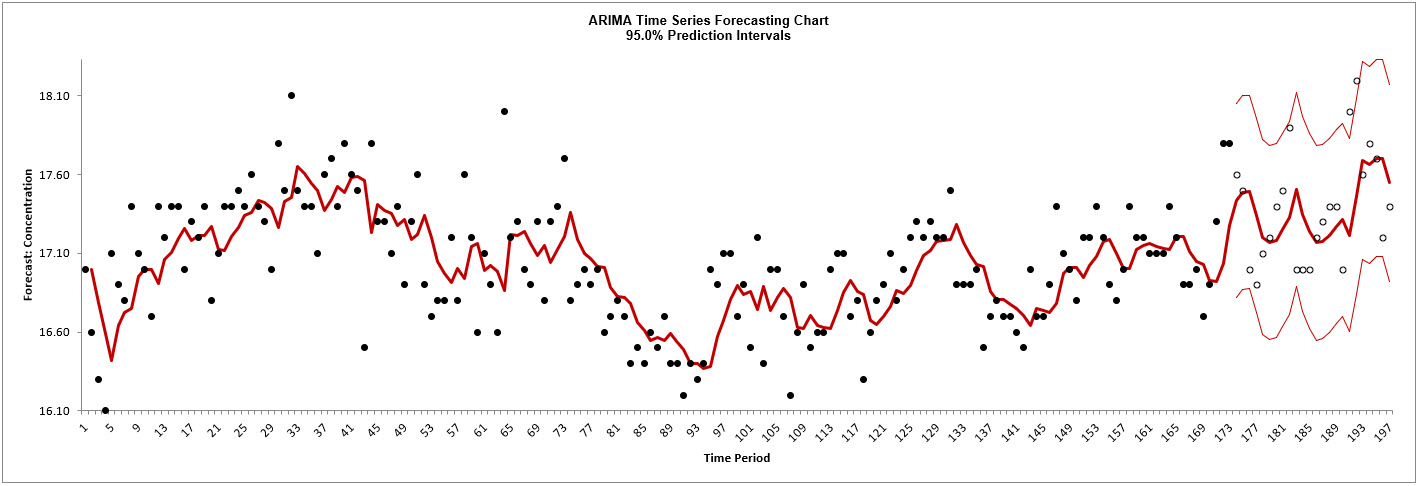The blank dots are the data values in the withhold sample with a one-step-ahead forecast and prediction intervals displayed at the start of the withhold sample.

This is very similar to the exponential smoothing forecast chart that was produced using the Simple Exponential Smoothing with Multiplicative Errors (M, N, N) model. The initial in-sample predicted value for ARIMA is slightly different and starts at the second time period due to differencing.

18. Scroll down to view the ARIMA Model header:19. The ARIMA Model Summary is given as: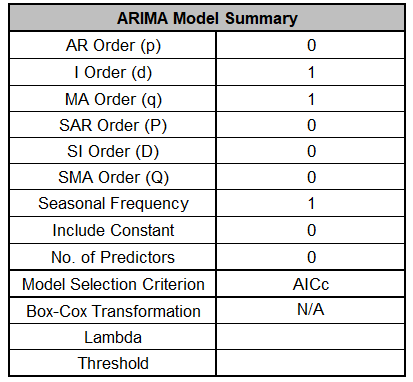The ARIMA (0,1,1) model that we manually specified above, was also automatically selected based on the AICc criterion.

20. The Parameter Estimates are:This is close to the parameter estimate obtained above (which used all of the data).

21. The ARIMA Model Statistics are: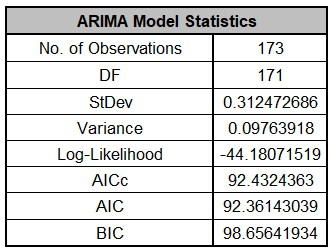These are fairly close to the model statistics obtained above using all of the data. Here we are using only 173 of the 197 observations.

22. The Forecast Accuracy metrics are: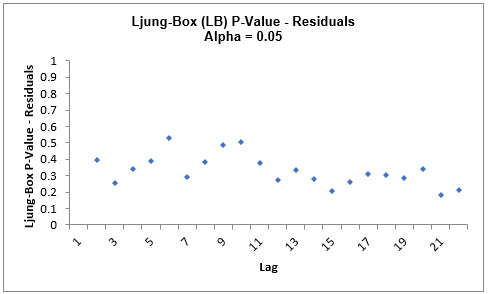As expected, the Out-of-Sample (Withhold) One-Step-Ahead Forecast errors are larger than the In-Sample (Estimation) One-Step-Ahead Forecast errors.

These are very similar to the Exponential Smooth Forecast Accuracy Metrics that were produced using the Simple Exponential Smoothing with Multiplicative Errors (M, N, N) model. Note that we lose one observation on the In-Sample (N=172) since we do not have a predicted value at time period = 1 due to differencing.

23. The Forecast Table is given as:These are the same forecast and prediction interval values displayed in the Forecast Chart, but provided for further analysis or charting. The Withhold Data is also displayed.

24. The ACF/PACF/LB Residual Plots and Residual Plots are based on the in-sample data. The plots look similar to the complete data above, except for the Ljung-Box P-Values:As was the case with exponential smoothing, the ARIMA (0,1,1) model is a better fit to the subset than the complete data, with all P-Values being blue (> .05).

25. If Include in Residuals was checked then the residuals would also include the Out-of-Sample (Withhold) One-Step-Ahead Forecast errors.
26. The above analysis can be rerun using Withhold Forecast Type: Multi-Step-Ahead with Prediction Interval at Start of Withhold (click Recall SigmaXL Dialog menu or press F3 to recall last dialog), but we will not do so here. The results would be very similar to those obtained with exponential smoothing.

The Autoregressive Integrated Moving Average (ARIMA) model was developed by Box and Jenkins. The default automatic determination of the best model order in SigmaXL uses the stepwise method of Hyndman and Khandakar (see fpp2).

Stationarity, Differencing and Constant

ARIMA assumes that the time series is stationary, i.e., it has the property that the mean, variance and autocorrelation structure do not change over time. If a time series mean is not stationary (e.g. trending), this can be corrected by differencing, computing the differences between consecutive observations for nonseasonal and between consecutive periods for seasonal data (e.g., Jan 2019 – Jan 2018, etc.). For nonseasonal, this will typically involve 1 or 2 orders of differencing. This order is the Integrated term d. For seasonal, this will typically involve 1 order of differencing. This order is the Seasonal Integrated term D. A constant term c is optional:

If d+D=0, a constant term in the model is the mean.
If d+D=1, a constant term in the model is a trend (drift).
If d+D>1, a constant term would be a quadratic or higher trend, so constant should not be included.
It is recommended that d+D should not be > 3.
If the variance is not stationary, use a Box-Cox transformation.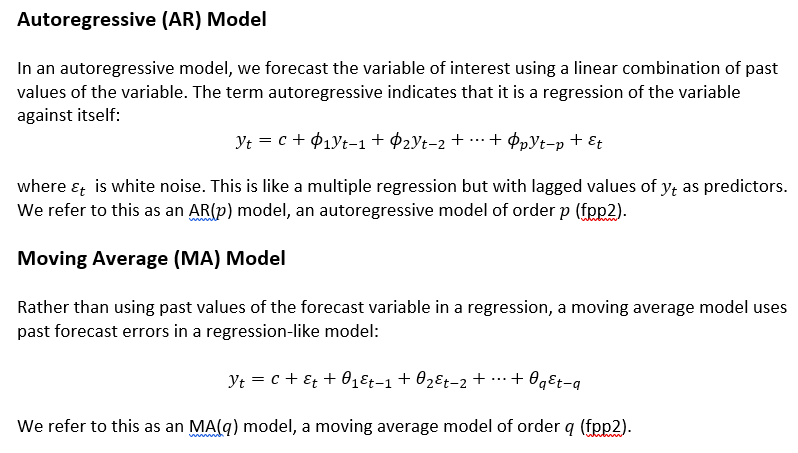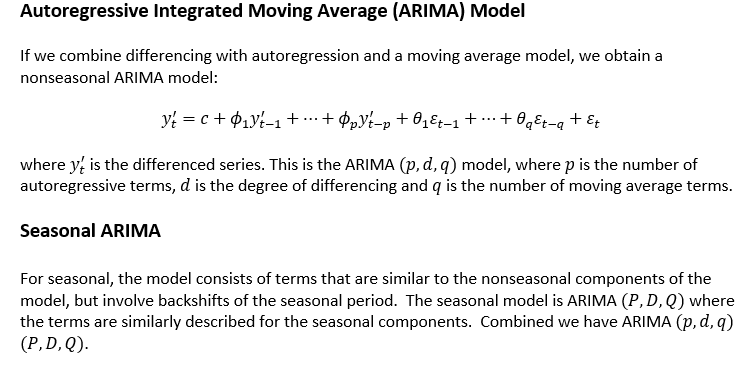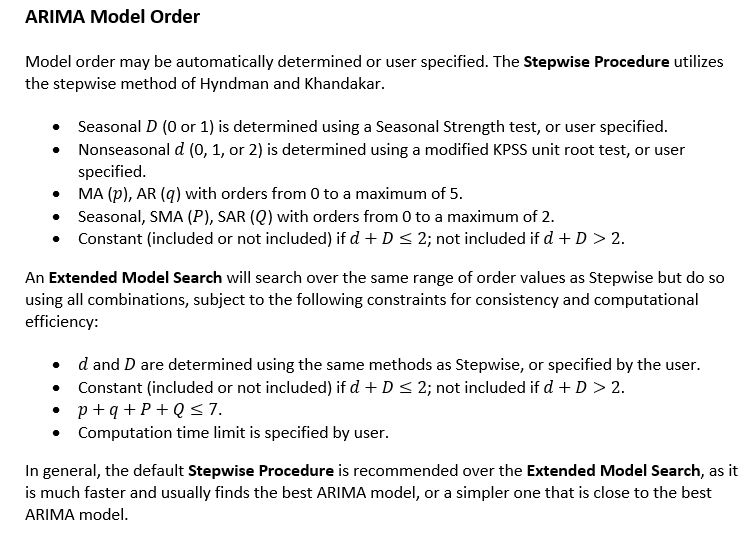Model Parameter Estimation and Missing Values

Model parameters are solved using nonlinear maximization of the Log-Likelihood function. Two general models are available - the conditional sum of squares (CSS) and the state space Kalman maximum likelihood. The CSS is always used for initial estimates and is used if n>500 or seasonal frequency >12 for computational efficiency. Kalman Filters permit exact calculations and can handle missing values. For CSS, if missing values are encountered, the largest contiguous range is used.

ARIMA Model Statistics and Information Criteria for Model Comparison

The ARIMA model statistics are similar to those used in Exponential Smoothing. Log-Likelihood is related to -Ln(Sum-of-Squares Error), so is maximized. Information Criteria AICc, AIC and BIC are calculated using -2*Log-Likelihood and incorporate a penalty for the number of terms in the model, so smaller is better. These are used in automatic model selection. AICc is the default Information Criterion, based on forecast error performance with competition data.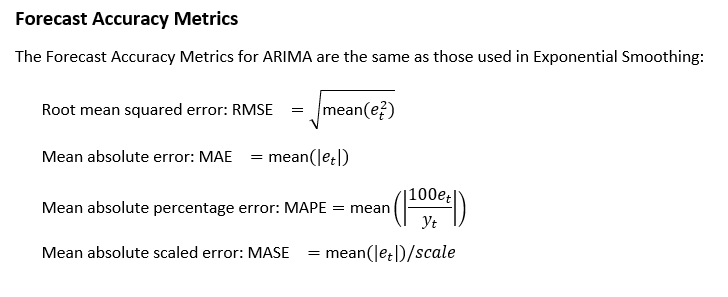Web Demos

Our CTO and Co-Founder, John Noguera, regularly hosts free Web Demos featuring SigmaXL and DiscoverSim